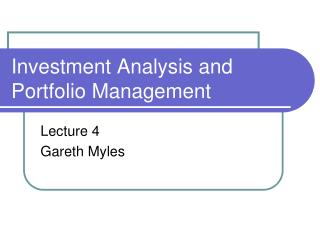DownloadDownload PresentationInvestment Analysis and Portfolio Management

# Investment Analysis and Portfolio Management

Télécharger la présentation## Investment Analysis and Portfolio Management

- - - - - - - - - - - - - - - - - - - - - - - - - - - E N D - - - - - - - - - - - - - - - - - - - - - - - - - - -
##### Presentation Transcript

1. Investment Analysis and Portfolio Management Lecture 4 Gareth Myles

2. Efficiency • An efficient investor will choose the portfolio with the maximum return for a given level of risk • Or the minimum risk for a given return • Any other choice is inefficient • To explore this idea it is necessary to determine the frontier of efficient portfolios • The set of portfolios with maximum return for given risk

3. Two-Asset Portfolios • Consider a portfolio composed of two assets A and B • The expected return on the portfolio is • The standard deviation of return is • The relationship between return and standard deviation is now derived

4. No Short Sales • Assume initially that there are no short sales • The holdings of both assets must be non-negative • This implies the asset proportions satisfy • Now consider the standard deviation for various values of the correlation coefficient

5. Perfect Positive Correlation • Case 1: Perfect positive correlation • The standard deviation becomes • The term in the bracket is a perfect square

6. No Short Sales • Taking the square root • The return is • This pair of equations determine a linear relation between expected return and standard deviation • The trade-off between risk and return is described by a straight line

7. Perfect Positive Correlation • An example • This gives the linear relations

8. Perfect Positive Correlation (XA = 1, XB= 0) A 14 8 B (XA = 0, XB= 1) 6 3

9. Perfect Negative Correlation • Case 2: Perfect negative correlation • This equation has two solutions 1. 2. • For the example

10. Perfect Negative Correlation • Notice that at • The existence of a portfolio with zero risk is a general property when • Notice also that • So

11. Perfect Negative Correlation Efficient (XA = 1, XB= 0) A 14 8 B (XA = 0, XB= 1) Inefficient 3 6

12. Intermediate Values • Case 3: • In the intermediate case the frontier must lie between that for the two extremes • It must be curved with no straight segments

13. Intermediate Values Efficient A (XA = 1, XB= 0) 14 Minimum variance portfolio 8 B (XA = 0, XB= 1) Inefficient 3 6

14. Minimum Variance Portfolio • Note that for every value of there is a portfolio with minimum variance • This is the one furthest to the left. • It is found be choosing to minimize • Taking the first-order condition and solving gives

15. An Example

16. An Example _ rp sp

17. The Efficient Frontier • The efficient frontier is the set of portfolios that have a higher return than the minimum variance portfolio • With additional assets the frontier is traced out be considering all possible portfolios • Any portfolio below the frontier is dominated by one on the frontier • There are no portfolios that allow risk/return combinations above the efficient frontier • The frontier is always concave

18. The Efficient Frontier Efficient A 14 C 8 B Inefficient 6 3

19. Allowing Short Sales • With short sales, no restrictions are placed on the proportions and except that • Allowing short selling introduces negative proportions • This extends the frontier at both ends • It extends indefinitely

20. Allowing Short Sales XA > 1, XB < 0 XA = 1, XB = 0 14 XA = 0, XB = 1 8 XA < 0, XB > 1 6 3

21. Riskfree Borrowing and Lending • Consider combining a portfolio, A, and the riskfree asset in proportions X and 1 - X • This gives expected return • And standard deviation • But and so

22. Riskfree Borrowing and Lending • Hence • Giving • The attainable risk and return combinations lie on a straight line

23. Risk-free Borrowing and Lending • Hence the efficient frontier is a straight line • It is tangential to the frontier without the risk-free asset c d b a

24. Risk-free Borrowing and Lending Efficient frontier Xf < 0, XT > 1 14 Xf = 0, XT = 1 Portfolio T: a mix of A and B 8 rf Xf = 1, XT = 0 6 3

25. Borrowing and Lending Rates • The above has assumed the interest rate for borrowing = interest rate for saving • In practice the borrowing rate is higher • Explained by asymmetric information • Borrowers other than governments cannot be treated as risk-free • Risky borrowers must pay a higher rate of interest • Borrowing rate rb > lending rate rf

26. Borrowing and Lending Rates • Efficient frontier now in three sections Efficient frontier XA > 1, XB < 0 14 XA = 1, XB = 0 rb XA = 0, XB = 1 8 rf XA < 0, XB > 1 3 6